# HSSlive: Plus One & Plus Two Notes & Solutions for Kerala State Board

## AP Board Class 7 Maths Chapter 11 Area of Plane Figures Ex 11.1 Textbook Solutions PDF: Download Andhra Pradesh Board STD 7th Maths Chapter 11 Area of Plane Figures Ex 11.1 Book AnswersAP Board Class 7 Maths Chapter 11 Area of Plane Figures Ex 11.1 Textbook Solutions PDF: Download Andhra Pradesh Board STD 7th Maths Chapter 11 Area of Plane Figures Ex 11.1 Book Answers

## Andhra Pradesh State Board Class 7th Maths Chapter 11 Area of Plane Figures Ex 11.1 Books Solutions

 Board AP Board Materials Textbook Solutions/Guide Format DOC/PDF Class 7th Subject Maths Chapters Maths Chapter 11 Area of Plane Figures Ex 11.1 Provider Hsslive

## How to download Andhra Pradesh Board Class 7th Maths Chapter 11 Area of Plane Figures Ex 11.1 Textbook Solutions Answers PDF Online?

2. Click on the Andhra Pradesh Board Class 7th Maths Chapter 11 Area of Plane Figures Ex 11.1 Answers.
3. Look for your Andhra Pradesh Board STD 7th Maths Chapter 11 Area of Plane Figures Ex 11.1 Textbooks PDF.
4. Now download or read the Andhra Pradesh Board Class 7th Maths Chapter 11 Area of Plane Figures Ex 11.1 Textbook Solutions for PDF Free.

## AP Board Class 7th Maths Chapter 11 Area of Plane Figures Ex 11.1 Textbooks Solutions with Answer PDF Download

Find below the list of all AP Board Class 7th Maths Chapter 11 Area of Plane Figures Ex 11.1 Textbook Solutions for PDF’s for you to download and prepare for the upcoming exams:

Question 1.
Calculate the area of the following triangles given below :
(a)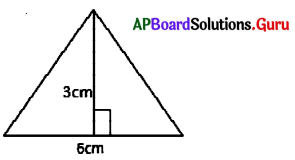From the figure, base = 6 cm
Height = 3 cm
Area of the triangle = 12 ∙ b ∙ h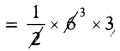= 9 sq.cm

(b)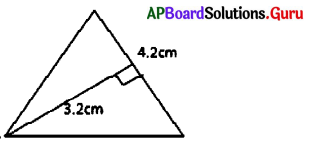From the figure, base = 4.2 cm
Height = 3.2 cm
Area of the triangle = 12 ∙ b ∙ h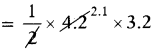= 6.72 sq.cm

(a)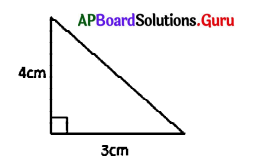From the figure, base = 3 cm
Height = 4 cm
Area of the triangle = 12 ∙ b ∙ h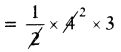= 6 sq.cm

(a)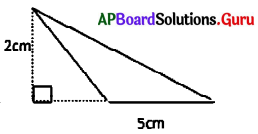From the figure, base = 5 cm
Height = 2 cm
Area of the triangle = 12 ∙ b ∙ h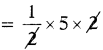= 5 sq.cm

Question 2.
Find the area of triangle with base 3.8 cm and height 4.6 cm.
Given base of the triangle b = 3.8 cm
Height of the triangle h = 4.6 cm
Area of the triangle = 12 ∙ b ∙ h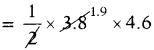∴ Area of the triangle = 8.74 sq.cm.

Question 3.
The surface area of a triangular shape window is 24 sq.m, and height is 6m., then find the base of the triangle, if the cost of glass fitting per sqm is ₹ 250, then find total cost of glass fitting for window.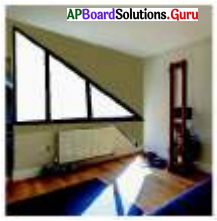Let,
base of the triangular window = b m
Height of the triangular window h= 6m
Area of triangular window = 12 ∙ b ∙ h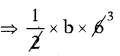= 24 sq.cm
⇒ 3b = 24
⇒ b = 243
= 8
∴ Base of the triangular window = 8m
Cost of glass fitting per sq.m = ₹ 250
Cost of glass fitting per 24 sq.m = 250 × 24
∴ Total cost of glass fitting for window = ₹ 6000/-

Question 4.
A traffic signal plate in the shape of triangle is having base 20 cm, height 15 cm. Find the area of the triangle. If the cost of painting is ₹ 2 per Sq.cm, then find the total cost for painting the signal board on one side.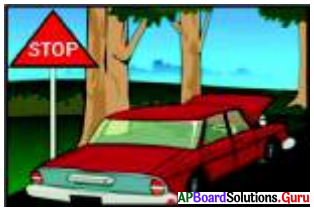Given the base of the signal plate b = 20 cm
Height of the signal plate h = 15 cm
Area of the signal plate = 12 ∙ b ∙ h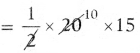= 150 sq.cm
∴ Area of the signal plate = 150 sq.cm
Cost of painting per sq.cm = ₹ 2
Cost of painting for 150 sq.cm = 150 × 2
∴ Total cost for painting the signal board = ₹ 300

Question 5.
find the area of triangular shaped wall painting whose base is 24m, height is 38m. Find the area of the triangle, if the cost of painting is ₹ 50 per Sq. m. and also find the total cost of painting.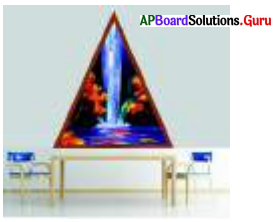Given base of the triangular wall painting = 24 m Height = 38 m
Area of wall painting = 12 ∙ b ∙ h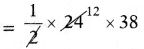= 456 sq.m.
Cost of painting per 1 sq.m = ₹ 50
Cost of painting for 456 sq.m = 456 × 50
∴ Total cost for painting per 456 sq.m = ₹ 22,800

Question 6.
The area of triangle shaped elevation of a house is 195 Sq.m. Its base is 26m. Find the height of elevation. Find the total cost of cementing, if the cost of cementing per Sq.m, is ₹ 250.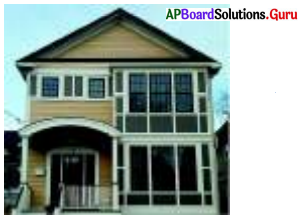Given, base of triangle shaped elevation of house b = 26 m height h = ?
Area of the triangular elevation = 195 sq.m
12 ∙ b ∙ h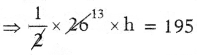⇒ 13 h = 195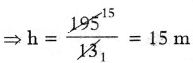Height of triangular elevation = 15 m
Cost of cementing per sq.m = ₹ 250
Cost of cementing per 195 sq.m
= 195 × 250
∴ Total cost of cementing of triangular elevation = ₹ 48,750

## Andhra Pradesh Board Class 7th Maths Chapter 11 Area of Plane Figures Ex 11.1 Textbooks for Exam Preparations

Andhra Pradesh Board Class 7th Maths Chapter 11 Area of Plane Figures Ex 11.1 Textbook Solutions can be of great help in your Andhra Pradesh Board Class 7th Maths Chapter 11 Area of Plane Figures Ex 11.1 exam preparation. The AP Board STD 7th Maths Chapter 11 Area of Plane Figures Ex 11.1 Textbooks study material, used with the English medium textbooks, can help you complete the entire Class 7th Maths Chapter 11 Area of Plane Figures Ex 11.1 Books State Board syllabus with maximum efficiency.

## FAQs Regarding Andhra Pradesh Board Class 7th Maths Chapter 11 Area of Plane Figures Ex 11.1 Textbook Solutions

#### Can we get a Andhra Pradesh State Board Book PDF for all Classes?

Yes you can get Andhra Pradesh Board Text Book PDF for all classes using the links provided in the above article.

## Important Terms

Andhra Pradesh Board Class 7th Maths Chapter 11 Area of Plane Figures Ex 11.1, AP Board Class 7th Maths Chapter 11 Area of Plane Figures Ex 11.1 Textbooks, Andhra Pradesh State Board Class 7th Maths Chapter 11 Area of Plane Figures Ex 11.1, Andhra Pradesh State Board Class 7th Maths Chapter 11 Area of Plane Figures Ex 11.1 Textbook solutions, AP Board Class 7th Maths Chapter 11 Area of Plane Figures Ex 11.1 Textbooks Solutions, Andhra Pradesh Board STD 7th Maths Chapter 11 Area of Plane Figures Ex 11.1, AP Board STD 7th Maths Chapter 11 Area of Plane Figures Ex 11.1 Textbooks, Andhra Pradesh State Board STD 7th Maths Chapter 11 Area of Plane Figures Ex 11.1, Andhra Pradesh State Board STD 7th Maths Chapter 11 Area of Plane Figures Ex 11.1 Textbook solutions, AP Board STD 7th Maths Chapter 11 Area of Plane Figures Ex 11.1 Textbooks Solutions,
Share: